Python简明教程——Python一小时快速入门

Python一小时快速入门 1.Python简介 pylogo.png Python是一种相当高级的解释性程序…

Python一小时快速入门

1.Python简介pylogo.png

Python是一种相当高级的解释性程序设计语言，完成同一个任务C语言可能需要几千行代码
Java要写100行，Python可能只需要20行。

Python当然也有不能干的事情，比如写操作系统，这个只能用C语言写；写手机应用，只能用Swift/Objective-C（针对iPhone）和Java（针对Android）；写3D游戏，最好用C或C++。

python提供了完善的内置库，覆盖了网络、文件、GUI、数据库、文本等大量内容，用python开发，很多功能不必从零编写，直接使用现成的即可。

python适合开发网络应用、日常小工具、科学计算等活动。

2.安装Python

Python有两个版本，2.x和3.x互不兼容，按需求安装即可。

python解释器：我们写的代码会在解释器上运行，类似JVM的机制，我们安装的标准解释器是用C编写的，称为CPython解释器，另外有IPython 是基于CPython交互解释器。还有Java写的Jpython解释器等等。我们一般使用Cpython。

3.python环境基本操作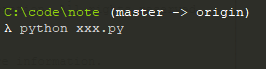prompt.png

python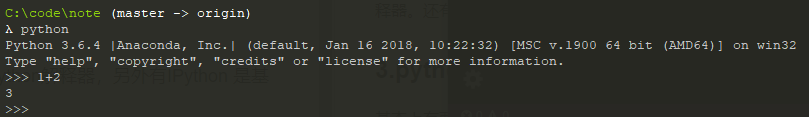interactive.png

print('Hello World')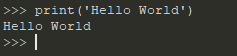helloworld.png

print('Hello World!n')

python hello.py
python xxx.py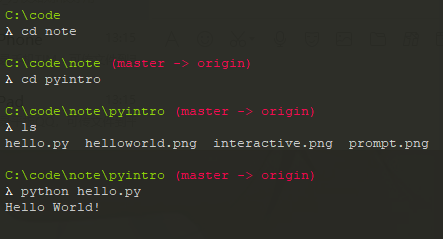excute.png

x=3.14
print(x)
mingzi='Mark'
print(mingzi)
print(300+500)
print('name')

print()也可以打印多个字符串

print(name,age,school)//用逗号隔开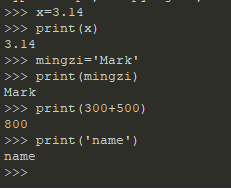x=input('请输入内容:')

print(x)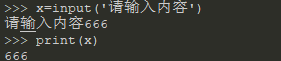name=input('请输入姓名:n')
print('你的名字是:'+name)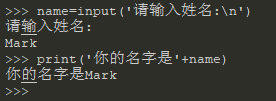4.python基础

语法

python的语法采取缩进语句块，不需要C、java的大括号区分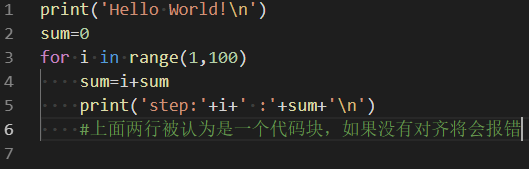#`＃`号后内容是注释

数据类型

python中所有数据类型都是对象，python创建一个数据类型不需要定义和提前声明，当一个变量赋值后就创建了它。

整数

python可以处理任意大小的整数，和matlab有异曲同工之妙，数据类型并没有固定的类型长度。

10,-100,214700000000000000等等

字符串

s='abcd'
len(s)=4
s='a'
s='d'

s='abcd'
s[-1]='d'
s[-2]='c'

s='abcd'
s[1:3]='bc'

s[:3]='abc'
s[1:]='bcd'
s[:]='abcd'

s+'zxyz='abcdxyz'
不可变性

s='abcd'
s='x'是错误的

n=s+'x'
python中，数字、字符串、元组都是不可变的。列表、和字典可变。
字符串类型特定方法

find()find方法是字符串特有的字串查找方法

s='china'
s.find('in')=3

replace()是替换字符串内字符的方法。

s.replace('in','out')='chouta'
s='china'//s并没有改变。

split()是分离方法

s='aaa,bbb,ccc'
s.split(',')=['aaa','bbb','ccc']

name='china'
dir(name)
help(name.split)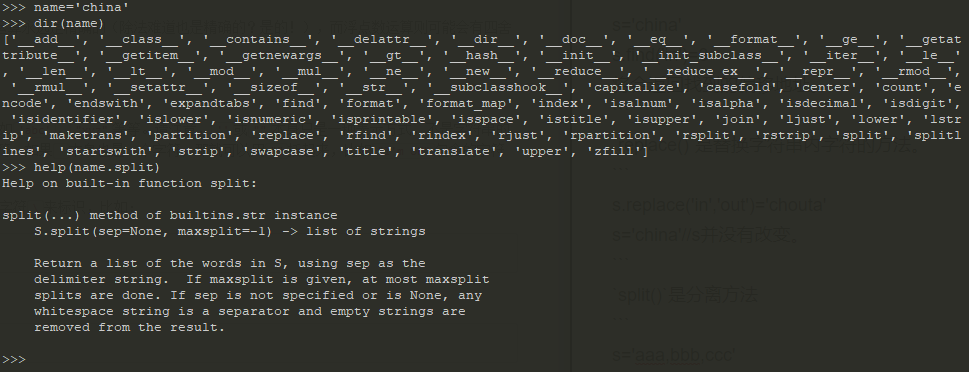help.png

str(数字)可以将数字转换为字符串，int（string）可以将字符串转换为数字等等

列表 list

s=['abc',123,3.14]
len(s)=3

s=123
s[:-1]=['abc',123]
s+[4,5,6]=['abc',123,3.14,4,5,6]
s=['abc',123,3.14]s仍然没有被改变
列表特定方法

append() 在列表末尾增加对象
pop()丢弃列表内容。
sort()列表排序,默认升序。
reverse()列表反转

s=[123,456,789]
s.append(666)
s=[123,456,789,666]
s.pop(2)
s=[123,456,666]
s.reverse()
s=[666,456,123]
s.sort()
s=[123,456,666]

m=[[1,2,3],[4,5,6],[7,8,9]]是一个3×3矩阵

m=[4,5,6]
列表解析

col=[row for row in m]
=[2,5,8]

col=[row+1 for row in m if row%2==0]
=[3,9]

G=(sum(row) for row in m)
next(G)=6
next(G)=15

map(sum,m）
=[6，15，24]
map(min,m)
=[1,4,7]

s=（1,2,3,4)
len(t)
=4

s.index(4)
=3

s.count(4)
=1

4只出现一次

字典 dict

D['name']='zhangsan'

D={'name': 'zhangsan','age': '20','job': ['dev','manager']}
D[job]
= ['dev','manager']
D[job][-1]
='manger'

D={b:'name',c:'job',a:'age',d:'gender;}

ks=list(D.keys())
ks.sort()
for key in keys:
print(key,'=>',D[key])

for key in sorted(D)
print(key,'=>'D[key]

循环

for循环自带迭代器，可以步进循环列表、字符串、对象等

for i in range(2,100,2):
x=sum(i)
print(sum)

for i in s:
print(i)

for i in l:
print(i)

for i in range(1,10):
print('*'*i+"n")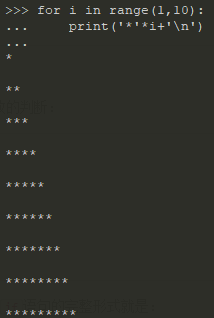yangtangle.png

while循环

x=4
while (x>4):
x--
print(x)

if-else语句

a=100,b=10
if a<b:
print(a)
elif a==b:
print(a,b)
else :
print(b)

break提前退出当前循环
continue跳过本次循环

函数

python中的函数非常简单

def Fun_Name (para1,para2)
if xxx
return xxx
else
xxx
return xxx

x=Fun_name(para1,para2)

def name()
pass

import math
def move(x,y,z,step,angle)
nx=x+step*math.cos(angle)
ny=y+step*math.sin(angle)
nz=z+step*math.tan(angle)
return nx,ny,nz

x,y,z=move(100,100,0,60,math.pi/3)
print(x,y,z)

role=move(100,100,0,60,math.pi/3)
print(role)

def funame (x,y=2)

funame(x)

def cal(numbers)
x=0
for n in numbers
x+=sum(n)
return (x)

def cal(*numbers)
x=0
for n in numbers
x+=sum(n)
return (x)

nums = [1, 2, 3]
calc(nums, nums, nums)
=6

nums = [1, 2, 3]
calc(*nums)
=6

def person(name, age, **kw):
print('name:', name, 'age:', age, 'other:', kw)

person('Michael', 30)
=name: Michael age: 30 other: {}

person('Bob', 35, city='Beijing')
=name: Bob age: 35 other: {'city': 'Beijing'}
=name: Adam age: 45 other: {'gender': 'M', 'job': 'Engineer'}

5.python进阶

import math

import xxx后python将会找到包所在地址，并且编译，开销很大，但之后就可以直接使用它的函数，xxx.function()

import random
ramdom.choice([1,2,3])

import random as ran
ran.choice([1,2,3])

from xxx import function（）
from random import choice()
choice([1,2,3])

math时python提供的模块（库）（包，我们可以使用自己写的模块，也可以使用第三方模块

conda install packagename